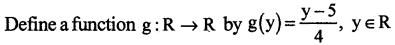# 2nd PUC Maths Question Bank Chapter 1 Relations and Functions

Students can Download 2nd PUC Maths Chapter 1 Relations and Functions Questions and Answers, Notes Pdf, 2nd PUC Maths Question Bank with Answers helps you to revise the complete Karnataka State Board Syllabus and to clear all their doubts, score well in final exams.

## Karnataka 2nd PUC Maths Question Bank Chapter 1 Relations and Functions

### 2nd PUC Maths Relations and Functions One Mark Questions and Answers

Question 1.
Give an example of a relation which is symmetric and transitive but not reflexive.
Let A = {a,b,c} Define a relation R on A by R = {(a,b),(b,a),(a,a),(b,b)} R is a relation which is symmetric and transitive but not reflexive because (c,c) ∉ R.

Question 2.
Give an example of a relation which is symmetric but neither reflexive nor transiitive.
Let A= {a,b,c} Define a relation R on A by R = {(a,b),(b,a)} R is a relation which is symmetric but neither reflexive nor transitive because (a, a) ∉ R.Question 3.
Give an example of a relation which is transitive but neither reflexive nor symmetric.
Let A = {a,b,c} Define a relation R on A by R = {(a,b),(b,c),(a,c)} R is a relation which is transitive but neither reflexive nor symmetric because (a,a) ∉ R and (b,a) ∉ R.

Question 4.
Give an example of a relation which is reflexive and symmetric but not transitive.
Let A = {a,b,c} Define a relation R on A by R = {(a,a),(b,b),(c,c)(b,c),(c,b),(a,b),(b,a)} R is a relation which is reflexive and symmetric but not transitive because (c, b) ∈ R and (b, a) ∈ R but (c,a) ∉ R.

Question 5.
Give an example of a relation which is reflexive and transitive but not symmetric.
Let A = {a,b,c} Define a relation R on A by R = {(a,a),(b,b),(c,c)(b,c),(c,b),(a,b)} R is a relation which is reflexive and transitive but not symmetric because (b, a) ∉ R.

Question 6.
Define an equivalence relation.
A relation R on a set A is said to be an equivalence relation if R is reflexive, symmetric and transitive.

Question 7.
Define a reflexive relation.
A relation R on a set A is said to be reflexive if (a, a) ∈ R, for every a ∈ A.

Question 8.
Define a symmetric relation.
A relation R on a set A is said to be symmetric if (a, b) ∈ R implies (b, a) ∈ R, a,b ∈ A.Question 9.
Define a transitive relation.
A relation R on a set A is said to be transitive if (a,b) ∈ R and (b,c) ∈ R implies (a,c) ∈ R a,b,c ∈ A.

Question 10.
Define an injective function.
A function f : A → B is said to be one – one (injective) if distinct elements of A have distinct images in B. i. e. for every x, y ∈ A, f(x) = f(y) implies x = y.

Question 11.
Define a surjective function.
A function f : A → B is said to be onto (surjective) if every element of B is the image of some element of A under f. i. e. for every y ∈ B, there exists an element x in A such that f(x) = y.

Question 12.
Define a bijective function.
A function f : A → B is said to be bijective if it is both one – one and onto.

Question 13.
If f : {1,3,4} → {1,2,5} and g: {1,2,5} → {1,3} are two functions given by f = {(1,2), (3,5), (4,1)} and g = {(1,3), (2,3), (5,1)}. Write down gof.
gof (1) = g (f (1) = g (2) = 3, gof (3) = gof (3)) = g (5) = 1
gof (4) = g (f (4)) = g (1) = 3, gof= {(1, 3) (3, 1), (4, 3)}

Question 14.
Let be a binary operation defined on the set of non-zero rational numbers, by a * b = $$\frac{a b}{4}$$. Find the identity element. (March 2015)
Let e be the identity element.
∴ a * e = a
$$\frac{a e}{4}$$ = a ⇒ e = 4.

Question 15.
a * b = ab ∀a,b ∈ Z. Show that × is not a binary operation on Z by giving a counter example.
Taking a = 2, b = – 3 we get a * b = 2 * -3 = 2-3 = $$\frac { 1 }{ 8 }$$ ∉ Z
∴ * is not a binary operation on Z.Question 16.
The operation defined by a * b = a. Is * a binary operation on z+.
a * b = a ∈ z+ ∀ a,b ∈ z+
∴ * is a binary operation on z+

Question 17.
Give an example of a relation which is symmetric only.
Let L be the set of all lines in a plane and R be the relation in L defined as R = {(L1, L2): L1 is perpendicular to L2}. R is symmetric only ∵ L1 ⊥ L2 ⇒ L1 ⊥ L2

Question 18.
Let * be a binary operation on N defined by a * b = LCM of a and b. Find 20 * 16.
20 * 16 = LCM of 20 and 16 = 80

Question 19.
Is * defined on the set A = {1,2,3,4,5} by a * b = LCM of a and b. Binary operation on A. Justify your answer.
2 * 3 = LCM of 2 and 3 – 6 ∉ A
∴ * is not a binary operation on A.

Question 20.
Let * be defined on the set on A = {1,2,3,4,5} by a * b = HCF of a and b. Is * a binary operation on A.
Yes ∵ a * b = HCF of a and b ∈ A ∀ a,b ∈ A
∴ * is a binary operation on A

Question 21.
Let * be a Binary operation on the set Q of Rational numbers by a * b = (a – b)2. Prove that is commutative.
a * b = (a – b)2 = (b – a)2 = b * a ∈ Q a,b ∈ Q

Question 22.
Let * be a Binary operation defined on N by a * b = 2ab. Prove that * is Commutative.
a * b = 2ab = 2ba = b * a v a,b ∈ N
∴ * is commutative.Question 23.
Let * be a Binary operation on N defined by a * b = ab2. Show that * is not commutative by giving a counter example.
2 * 3 = 2.32 = 2.9 =18
3 * 2 = 3.22 = 3.4 = 12
∴ 2 * 3 ≠ 3 * 2
∴ * is not commutative

Question 24.
Show that * : R × R → R defined by a * b = a + 2b is not commutative in R.
3 * 4 = 3 + 2 (4) = 3 + 8 = 11
4 * 3 = 4 + 2(3) = 4 + 6 = 10
3 * 4 ≠ 4 * 3
∴ * is not commutative

Question 25.
Show that * :R × R → R defined by a * b = a + 2b is not associative in R.
(8 * 5) * 3 = (8 + 10) * 3 = 18 * 3 = 18 + 2(3) = 24 .
8 * (5 * 3) = 8 * (5 + 2(3)) = 8 * (5 + 6)
= 8 * 11 = 8 + 2(11)
= 8 + 22
= 30 * 5) * 3) ≠ 8 * (5 * 3)
∴ * is not associative.

Question 26.
Show that the binary operation * defmed on R by a * b = $$\frac{\mathbf{a}+\mathbf{b}}{\mathbf{2}}$$ is not associative by giving a counter example.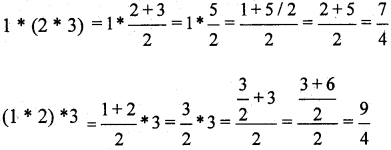∴ (1 * (2 * 3) ≠ (1 * 2) * 3)
∴ * is not associative.

Question 27.
Show that the operation * defined on R by a * b = 1 ∀ a, b ∈ R is associative.
a * (b * c) = a * 1 = 1
(a * b) * c = 1 * c = 1
a * (b * c) = (a * b) * c
∴ * is associative

Question 28.
If f = {(5,2), (6,3)}, g = {(2,5), (3,6)} Write fog.
fog = {(2,2), (3,3)}Question 29.
Let R be the equivalence relation on z defined by R = {(a,b): 2 divides a – b}. Write the equivalence class 
 = {0, ± 2, ± 4, ± 6,…}

Question 30.
Let f: R → R be a function defined by f(x) = 4x – 3 ∀ x ∈ R. Then Write f-1
Let f-1(x) – y ⇒ f(y) = x
⇒ 4(y) – 3 = x
⇒ 4(y) = x + 3.
⇒ y = $$\frac{x+3}{4}$$
⇒ f-1 = $$\frac{x+3}{4}$$

### 2nd PUC Maths Relations and Functions Two Marks Questions and Answers

Question 1.
Define binary operation on a set. Verify whether the operation * defined on Z, by a × b = ab + 1 is binary or not.
A binary operation * on a set A is defined as a function *: A × A → A.
a * b = ab + 1 ∈ Z, ∀ a, b ∈ Z
∴ * is a binary operation on Z.

Question 2.
Show that the signum function f : R → R given byDomain of f is R, co-domain of f is R and Range of f = {-1, 0, 1}
f(2) = 1, f(3) = 1 ∴ f(2) = f(3) but 2 ≠ 3 ∴ f is not one-one.
Range of f ≠ codomain of f ∴ f is not onto
∴ f is neither one-one nor onto.Question 3.
A relation R is defined on the set A = {1, 2, 3, 4, 5, 6} by R = {(x, y) : y is divisible by JC}. verify whether R is symmetric and reflexive or not. Give reason. ,
R = {(1,1), (2,2), (3,3) (4,4), (5, 5), (6,6) (1,2), (1,3), (1,4), (1, 5), (1, 6), (2, 4), (2, 6), (3, 6)}. As (a, a) ∈ R ∀ a ∈ A ⇒ R is reflexive.
(1,2) ∈ R but (2,1) ∉ R ⇒ R is not symmetric.
Let (a,b) and (b,c) ∈ R ⇒ b is divisible by a and c is divisible by b ⇒ c is divisible by a ⇒ (a, c) ∈ R ⇒ R is transitive.

Question 4.
Prove that the greatest integer function, f : R → R defined by f(x) = [x], where [x] indicates the greatest integer not greater than x, is neither one-one nor onto.
Domain of f = R, co-domain of f = R and Range of f = Z
f(1.1) = 1, f(1.2) = 1 ∴ f(1.1) = f(1.2) but 1.1 ≠ 1.2 ∴ f is not one-one.
Range of f ≠ co-domain of f ∴ f is not onto.
∴ f is neither one-one nor onto.

Question 5.
Show that the function f : R → R, defined by f(x) = $$\frac{1}{x}$$ is one – one and onto where R is the set of all non-zero real numbers.
Let x,y ∈ R, such that f(x) = f(y) ⇒ $$\frac{1}{x}=\frac{1}{y}$$ ⇒ x = y ∴ f is one – one.
Let y ∈ R (codomain) then there exists x ∈ R, (domain) such that f (x) = y
⇒ $$\frac{1}{x}$$ = y or x = $$\frac{1}{y}$$ ∴ $$f\left(\frac{1}{y}\right)$$ = y ∀ y ∈ R. (co – domain) ∴ f is onto
f is both one-one and onto.

Question 6.
Show that the Modulus function f : R → R, given by f(x) = |x| is neither one-one nor onto.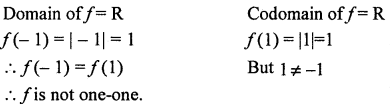-1 ∈ R (codomain) then there does not exist x∈R (domain)
such that f(x) = – 1
In fact, all negative integers do not have pre-image
Range of f = [0,∞)
∴ Range off ≠ co-domain of f
∴ f is not onto
∴ f is neither one-one nor onto.Question 7.
Show that the function f : N → N defined by f(x) = x2,∀ x ∈ N is injective but not surjective.
Domain of f = N Codomain of f = N
∀x1, x2 ∈ N, f (x1) = f(x2) ⇒ x12 = x22 ⇒ (x1 – x2 )(x1 + x2) ≠ 0
⇒x12 – x22 = 0 ⇒ x1 = x2 ⇒ f is one-one, x1, x2 ∈ N(x1 + x2) ≠ 0
Range of f = {12, 22, 32…….. } = {1, 4, 9} ≠ N (codomain) ∴ f is not onto.

Question 8.
Show that the function f : Z → Z defined by f(x) = x2 ∀ x ∈ Z is neither injective nor surjective.
∀x1, x2 ∈ Z, f(x1) = f(x2) ⇒ x12 = x22 ⇒ x2 = ±x1 ⇒ f is not one-one.
Range, of f = {02,(±1)2, (±2)2, ….} = {0,1,4…..} ≠ Z (codomain)
∴ f is not onto.

Question 9.
Show that the function f : R → R defined by f(x) = x2 ∀x∈R is neither injective nor subjective.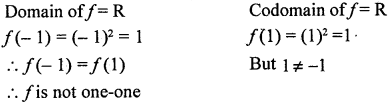-1 ∈ R (codomain) then there does not exist x ∈ R (domain)
such that f(x) = – 1
In fact, all negative integers do not have pre-image
Range of f = [0, ∞)
∴ Range of f ≠ co-domain of f
∴ f is not onto
∴ f is neither one-one nor onto.

Question 10.
Show that the function f : N → N defined by f(x) = x3 ∀x ∈ N is injective but not surjective.
Let f(x) = f(y) ⇒ x3 = y3 ⇒ x = y ∵ x,y ∈ N ∴ f isone-one.
Range of f = {13,23,33,…..} = {1, 8, 27,….} ≠ N (codomain)
∴ f is one-one but not onto.

Question 11.
Show that the function f : Z → Z defined by f(x) = x3 ∀ x ∈ Z is injective but not surjective.
∀ x,y ∈ Z, f(x) = f(y) ⇒ x3 = y3 ⇒ x = y f is one-one.
Range of f = {03, (±1)3, (±2)3, ….} = {0, ±1, ±8, } ≠ Z (codomain)
∴ f is not onto.Question 12.
Prove that the function f : R → R defined by f(x) = 2x, ∀x ∈ R is bijective.
f(x1) = f(x2) ⇒ 2x1 = 2x2 ⇒ x1 = x2 ∴ f is one-one.
Given any real number y in R there exists $$\frac{y}{2}$$ in R such that $$f\left(\frac{y}{2}\right)=2 \cdot\left(\frac{y}{2}\right)=y$$ ∴ f is onto.
∴ f is bijective

Question 13.
Prove that the function f : R → R defined by f(x) = 3 – 4x, ∀ x ∈ R is bijective.
f(x1) = f(x2) ⇒ 3 – 4x1 = 3 – 4x2 ⇒ x1 = x2 ⇒ f is one-one
Let y ∈ R, there exists x = $$\frac{3-y}{4}$$ ∈ R such that f(x) = $$f\left(\frac{3-y}{4}\right)=3-4\left(\frac{3-y}{4}\right)$$
= 3 – 3 + y = y ⇒ f is onto. ∴ f is one-one and onto ⇒ f is bijective.

Question 14.
A binary operation * on N defined as a * b = a3 + b3, show that * is commutative but not assossiative.
Let a,b,c ∈ N
Given, a * b = a3 + b3 = b3 + a3 = b × a ⇒ *is commutative.
Now, (a * b) * c = (a3 + b3) × c = (a3 + b3)3 + c3
And a * (b * c) = a * (b3 + c3) = a3 + (b3 + c3)3
Since (a3 + b3)3 + c3 ≠ a3 + (b3 + c3)3 ⇒ (a × b) × c ≠ a × (b × c)
∴ * is commutative but not assossiative.

Question 15.
A binary operation * on N defined as a × b = $$\sqrt{a^{2}+b^{2}}$$, show; that * is both commutative and associative.### 2nd PUC Maths Relations and Functions Three Marks Questions and Answers

Question 1.
Verify whether the function, f : A → B, where A = R – {3} and B = R – {1}, defined by f(x) = $$\frac{x-2}{x-3}$$ is one – one and on to or not . Give reason
Let x1, x2 ∈ A = R – {3} such that f(x1) = f(x2) ⇒ $$\frac{x_{1}-2}{x_{1}-3}=\frac{x_{2}-2}{x_{2}-3}$$
⇒ (x1 – 2) (x2 – 3) = (x1 – 3) (x2 – 2)
⇒ x1 x2 – 2x2 – 3x1 + 6
= x1x2 – 2x1 – 3x2 + 6
⇒ x2 = x1
∴ f is one – one.Question 2.
If * is a binary operation defined on A = N × N, by (a, b) * (c, d) = (a + c, b + d), prove that * is both commutative and associative. Find the identity if it exists.
Let {a, b), (c, d), (e, f) be any three elements of A = N × N
(a, b) * {(c, d) * (e, j)} = {a, b) * (c + e, d + f)
= ( a + c + e, b + d + f) = (a + c, b + d) * (e, f) = {(a, b) * (c, d)} * (e, f)}
∴ (A, *) is associative.
(a, b) * (c, d) = (a + c, b + d) = (c + a, d + b) = (c, d) * (a, b)
∴ (A, *) is commutative.
Let (x, y) be the identity element in A
∴ (a, b) * (x, y) = (a, b) ⇒ (a + x, b + y) = (a, b) ⇒ a + x = a, b + y = b
⇒ x = 0, y = 0 and 0 ∉ N ⇒ (x, y) = (0,0) ∉ A
∴ (A, *) does not have an identity element.Question 3.
Show that the relation R in the set of all integers, Z defined by R = {(a, b) : 2 divides a – b} is an equivalence relation.
2 divides 0 ⇒ 2 divides a – a ⇒ (a, a) ∈ R ∀ a ∈ Z ∴ R is reflexive.
Let (a,b) ∈ R ⇒ 2 divides a – b ⇒ 2 divides – (a – b)
⇒ 2 divides b – a ⇒ (b, a) ∈ R
∴ R is symmetric.
Let (a,b)) ∈ R, (b,c) ∈ R
⇒ 2 divides a – b and 2 divides b – c
⇒ 2 divides (a – b) + (b-c)
⇒ 2 divides a – c ⇒ (a, c) ∈ R is transitive.
∴ R is an equivalence relation.

Question 4.
Show that the relation R in the set of all natural numbers, N defined by is an R = {(a, b) : |a – b| is even} is an equivalence relation.
|a – a| = |0| = 0 is even ∴ (a, a) ∈ R ∴ R is reflexive.
Let (a,b) ∈ R ⇒ |a – b| is even ⇒ |-(a – b)| is even ⇒ |(b – a)| is even
⇒ (b,a) ∈ R ∴ R is symmetric.
Let (a,b) ∈ R and (b,c) ∈ R ⇒ | a – b| is even and |b – c| is even
⇒ a – b is even ⇒ b – c is even. ⇒ a – b + b – c is even
⇒ a – c is even ⇒ |a – c| is even. ∴ (a,c) ∈ R. ∴ R is transitive
∴ R is an equivalence relation.

Question 5.
Show that the relation R in| the set A = {x ∈ Z : 0 ≤ x ≤ 12} given by R = {{a, b) : |a – b| is a multiple of 4} is an equivalence relation.
A = {0, 1, 2, 3, 4, 5, 6 ,7, 8, 9, 10, 11, 12}
|a – a| = 0 divisible by 4 ⇒ (a,a) ∈ R ∀ a ∈ A ⇒ R is reflexive.
(a, b) ∈ R ⇒ | a – b | is divisible by 4 ⇒ |-(b -a)| is divisible by 4
⇒ | b – a| is divisible by 4 ⇒ (b, a) ∈ R ⇒ R is symmetric.
Let(a,b) and (b, c) ∈ R ⇒ |a – b| and |b – c| are divisible by 4.
⇒ a – b and b – c are divisible by 4 ⇒ (a – b) + (b – c) is divisible by 4
⇒ a – c is divisible by 4 ⇒ |a – c| is divisible by 4 ⇒ (a,c) ⇒ R
⇒ R is transitive.
Hence R is an equivalence relation.

Question 6.
Let R be relation on the set A = {1,2,3,… 14} by R = {(x, y): 3x – y = 0}. Verify R is reflexive symmetric and transitive. (March 2015)
R = {(1,3), (2,6), (3,9), (4,12)}
(1,1) ∉ R
∴ R is not reflexive (1,3) ∉ R But (3,1) ∉ R
∴ R is not symmetric (1,3) ∉ R, (3,9) ∉ R But (1,9) ∉ R
∴ R is not transitive.Question 7.
Relation R on Z defined as R = {(x,y): x – y is an integer}. Show that R is an equivalence relation
O is an integer
x – x is an integer
∴ (x, x) ∈ R ∀ x ∈ z
∴ R is reflexive
Let (x, y) ∈ R ⇒ x – y is an integer
⇒ -(x – y) is an integer
⇒ y – x is an integer
⇒ (y, x) ∈ R
∴ R is symmetric
Let (x, y) ∈ R and (y, z) ∈ R
⇒ x – y is an integer and y – z is an integer
⇒ (x – y) +(y – z) is an integer
⇒ x – z is an integer
⇒ (x, z) ∈ R
∴ R is transitive
∴ R is an equivalence relation

Question 8.
Show that the relation R in R defined by R = {(a, b): a ≤ b} is reflexive and transitive but not symmetric.
a ≤ a is always true
∴ (a, a) R a R
∴ R is reflexive
Let (a, b) ∈ R ⇒ a ≤ b which does not imply b ≤a
∴ (b, a) ∉ R
∴ R is not symmetric
Let (a, b) ∈ R and (b, c) ∈ R
⇒ a ≤ b and b ≤ c
⇒ a ≤ c
⇒ (a, c) ∈ R
∴ R is transitiveQuestion 9.
Show that if f: R – $$\left\{\frac{7}{5}\right\}$$ → R – $$\left\{\frac{3}{5}\right\}$$ is defined by f(x) = $$\frac{3 x+4}{5 x-7}$$ and g : R- $$\left\{\frac{3}{5}\right\}$$ → R – $$\left\{\frac{7}{5}\right\}$$ is defined by g(x) = $$\frac{7 x+4}{5 x-3}$$ then fog = IA and gof = IB, where, A = R- $$\left\{\frac{3}{5}\right\}$$,B = R – $$\left\{\frac{7}{5}\right\}$$; IA(X) = x, ∀ x ∈ A,IB(x) = x, ∀ X ∈ B are called identity functions on sets A and B, respectivelyThus, gof(x) = x, ∀ x ∈ B and fog(x) = x, ∀ x ∈ A, which implies that gof = IB and fog = IA

Question 10.
Show that if f : A → B and g : B → C are one-one, then gof : A → C is also one-one.
Suppose gof(x1) = gof(x2)
⇒ g(f(x1)) = g(f(x2))
⇒ f(x1) = f(X2), as g is one-one
⇒ x1 = x2, as f is one-one.
Hence, gof is one-one.

Question 11.
Show that iff: A → B and g: B → C are onto, then gof : A → C is also onto.
Given an arbitrary element z – C, there exists a pre-image y of z under g such that g(y) = z i. e., g is onto. Further, for y ∈ B, there exists an element x in A with f(x) = y, since f is onto. Therefore, gof(x) = g(f(x)) = g(y) = z, showing that gof is onto.

Question 12.
Consider f: N → N,g: N → N and h: N → R defined as f(x) = 2x, g(y) = 3y+4 and h(z) = sin z, ∀ x, y and z in N. Show that ho(gof) = (hog) of.
We have ,
ho(gof)(x) = h(gof(x)) = h(g(fx))) = h(g(2x))
= h(3(2x) + 4) = h(6x + 4) = sin(6x + 4) ∀ x ∈ N.
Also, ((hog)of)(x) = (hog) (f(x)) + (hog) (2x) h(g(2x))
= h(3(2x) + 4) = h(6x + 4) = sin(6x + 4), ∀ x ∈ N.
This shows that ho(gof) = (hog) of.

Question 13.
If f: X → y, g: Y → 3 z and h: Z → S are functions, then ho(gof) = (hog)of.
Proof: We have
ho(gof) (x) = h(gofx)) = h(g(f(x))), ∀ x in X
and (hog) of (x) = hog(gx)) = h(g(f(x))), ∀ x ¡n X
Hence, ho(gof) = (hog) of.Question 14.
Let f: X → Y and g: Y → Z be two invertible functions. Then gof is also invertible with (gof)-1 = f-1og-1.
Proof: To show that gof is invertible with (gof)-1 = f-1og-1, it is enough to show that (f-1og-1)o(gof) = Ix and (gof)o(f-1og-1) = Iz
Now, (f-1og-1)o(gof) = ((f-1og-1)og) of, by Theorem 1
= ((f-1o(g-1og) of, by Theorem I
= ((f-1oIY) of, by definition of g-1
= Ix.
Similarly, it can be shown that (gof)o(f-1og-1) = Iz.

Question 15.
If R1 and R2 are equivalence relations in a set A, show that R1 ∩ R2 is also an equivalence relation.
Since R1 and R2 are equivalence relations, (a, a) ∈ R1, and (a, a) ∈ R2 ∀ a ∈ A. This implies that (a,a) ∈ R1 ∩ R2, ∀ a, showing R1 ∩ R2 is reflexive. Further, (a,b) ∈ R1 ∩ R2 ⇒ (a,b) ∈ R1 and (a,b) ∈ R2 ⇒ (b,a) ∈ R2 and (b,a) ∈ R2 ⇒ (b,a) ∈ R1 ∩ R2, hence R1 ∩ R2 is symmetric. Similarly, (a, b) ∈ R1 ∩ R2 and (b, c) ∈ R1 ∩ R2 ⇒ (a, c) ∈ R1 and (a, c) ∈ R2 ⇒ (a, c) ∈ R1 ∩ R2. This shows that R1 ∩ R2 is transitive. Thus, R1 ∩ R2 is and equivalence relation.

Question 16.
Prove that the relation R defined on the set of real numbers R as
R = {(a,b) : a ≤ b2 ∀ a,b ∈ R} is neither reflexive nor symmetric nor transitive.
$$\left(\frac{1}{2}, \frac{1}{2}\right)$$ ∉ R since $$\frac{1}{2} \leq\left(\frac{1}{2}\right)^{2}$$ is not true .
∴ R is not reflexive.
(1,4) ∈ R ∵ 1 ≤ 42 is true. ∴ R is not symmetric.
But(4,1) ∉ R. (3,2),(2,1.5) ∈ R But(3, 1.5) ∉ R. ∴R is not transitive.

### 2nd PUC Maths Relations and Functions Six Marks Questions and Answers

Question 1.
Prove that the function f : N → Ydefined by f(x) = x2, where Y = {y : y = x2, x ∈ N} is invertible. Also write the inverse off (x). (June 2014)
Let f(x1) = f(x2) ⇒ x12 = x22 ⇒ x1 = x2(∵ x1, x2 ∈ N) ∴ f is one – one.
Domain of f = N, codomain of f = Y
Range of f = {12, 22, 32, ……..} {1, 4,9 ………..} codornain of f
∴ f is onto. ∴ f is bijective. ∴ f-1 exists.
An arbitrary element y in Y is of the form x2, for some X ∈ N x ⇒ √y
This gives a function g : Y → N, defined by g(y) = √y
Now gof (x) = g(x2) = $$\sqrt{x^{2}}$$ = x and fog(y) = $$f(\sqrt{y})=(\sqrt{y})^{2}$$ = y.
⇒ gof = IN and fog = Iy Hence, f is invertible with f-1 = g.Question 2.
Let f : N → R be defined by f(x) = 4x2 + 12x + 15,show that f : N → S, where S is the range of the function, is invertible. Also find the inverse.
Let f(x1) = f(x2)
⇒ 4x12 + 12x1 + 15
⇒ 4x22 + 12x2 + 15
⇒ 4(x12 – x22) + 12(x1 – x2) = 0
⇒ 4(x1 – x2)(x1 + x2) + 12(x1 – x2) = 0
⇒ (x1 – x2) [(x1 + x2) + 12] = 0
⇒ (x1 – x2) = 0 (∵ 4((x1 + x2) +12 ≠ 0)
⇒ x1 = x2 ∴ f is one-one
For the function f : N → S, codomain of f = S = Range of f (given)
∴ f is onto. ∴ f is bijective. ∴ f-1 exists.
Let y be an arbitrary element of S. Then y = 4x2 + 12x + 15, for some x in
N ⇒ y(2x)2 + 2.2x.3 + 32 + 6 = (2x + 3)2 + 6. = (2x+3)2 = y – ó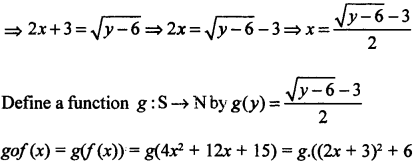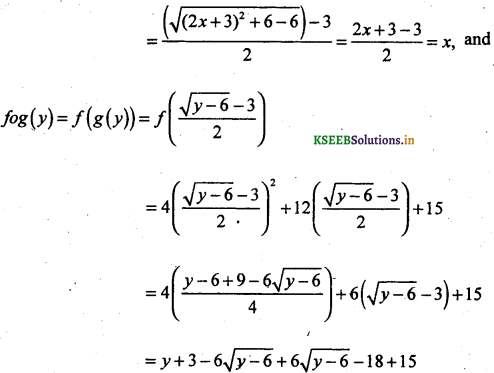Question 3.
Verify whether the function f : N → Y defined by f (x) = 4x + 3, where Y = {y : y = 4x + 3, x ∈ N} is invertible or not. Write the inverse of f(x) if exists. (March 2014)
Let f (x1) = f (x2)
⇒ 4x1 + 3 = 4x2 + 3
⇒ 4x1 = 4x2
⇒ x1 = x2
∴ f is one-one.
Let y ∈ Y ∃ X ∈ N such that y = 4x + 3 ∴ f is onto.
∴ f is bijective.
Consider an arbitrary element y of Y. By the definition of Y, y = 4x +3, for some x in the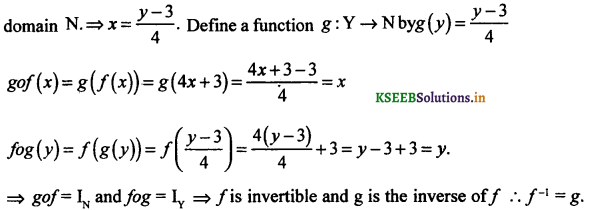Question 4.
Let R+ be the set of all non-negative real numbers. Show that the function f : R+ → [4,∞) defined by f(x) = x2 + 4 is invertible. Also write the inverse off. (March 2015)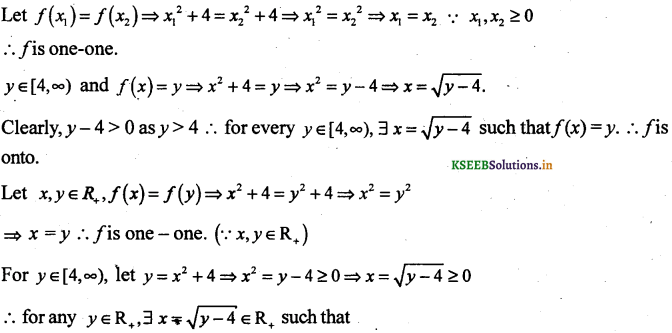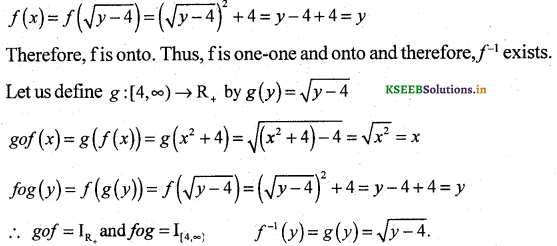Question 5.
If R+ is the set of all non-negative real numbers prove that the f : R+ → [-5,∞) defined byf(x) = 9x2 + 6x – 5 is invertible. Write also f-1 (x).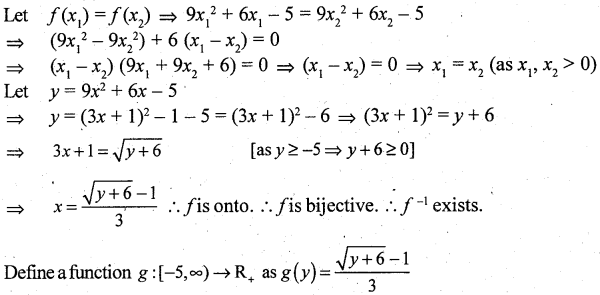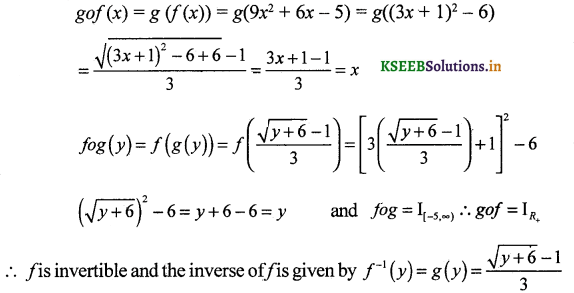Question 6.
If A → A where A = R – $$\left\{\frac{2}{3}\right\}$$ defined by f(x) = $$\frac{4 x+3}{6 x-4}$$ is invertible. Prove that f ‘f.
To Prove that f is one – one
f(x1) = f(x2)
$$\frac{4 x_{1}+3}{6 x_{1}-4}=\frac{4 x_{2}+3}{6 x_{2}-4}$$
(4x1 + 3)(6x2 – 4) = (4x2 + 3)(6x1 – 4)
24x1x2 – 16x1 + 18x2 – 12 = 24x1x2 – 16x2 + 18x1 – 12
-18x1 – 16x1 = -16x2 – 18x2
– 34x1 = – 34x2
∴ x1 = x2
∴ f is one-one
To prove that f is onto.
Let y ∈ A ∃ x ∈ A such that f(x) = y
$$\frac{4 x+3}{6 x-4}=y$$
4x + 3 = 6xy – 4y
4x – 6xy = -4y – 3
6xy – 4x = 4y + 3
x(6y – 4) = 4y + 3
x = $$\frac{4 y+3}{6 y-4}$$ ∈ A
∴ f is onto.
∴ f is invertible.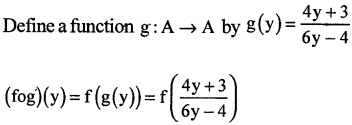Question 7.
f: R → R be defmed as f(x) = 4x + 5 ∀ X ∈ R show that fis invertible and find f-1 (June 2015)